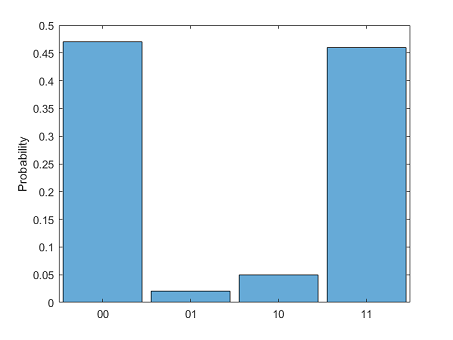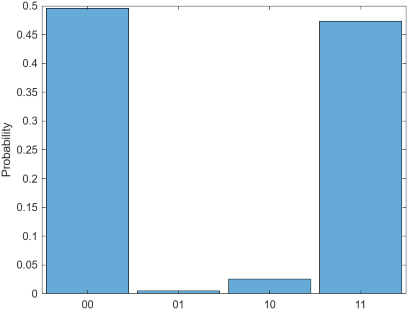# fetchOutput

Since R2023a

Installation Required: This functionality requires MATLAB Support Package for Quantum Computing.

## Syntax

``m = fetchOutput(task)``

## Description

example

````m = fetchOutput(task)` returns the measurement result of a finished quantum task as a `QuantumMeasurement` object.A measurement result is obtained only if the status of the task is `"finished"` and the task is not canceled. You can use `wait(task)` to make sure a task is finished before retrieving the measurement result using `fetchOutput`.```

## Examples

collapse all

Create a quantum circuit that consists of a Hadamard gate and a controlled X gate to entangle two qubits.

```gates = [hGate(1); cxGate(1,2)]; c = quantumCircuit(gates);```

Connect to a remote quantum device through AWS®. Create a task that runs the circuit on the device.

```dev = quantum.backend.QuantumDeviceAWS("Aspen-M-3"); task = run(c,dev)```
```task = QuantumTaskAWS with properties: TaskARN: "arn:aws:braket:us-west-1:123456789012:quantum-task/12a34b5c-6a78-9a01-2ab3-4c56def7g890" Status: "queued"```

Wait for the task to finish and retrieve the result.

```wait(task) m = fetchOutput(task)```
```m = QuantumMeasurement with properties: MeasuredStates: [4×1 string] Counts: [4×1 double] Probabilities: [4×1 double] NumQubits: 2```

Show the counts and estimated probabilities of the measured states.

```table(m.Counts,m.Probabilities,m.MeasuredStates, ... VariableNames=["Counts","Probabilities","States"])```
```ans = 4×3 table Counts Probabilities States ______ _____________ ______ 47 0.47 "00" 2 0.02 "10" 5 0.05 "01" 46 0.46 "11" ```

Plot these measured states as a histogram of estimated probabilities.

`histogram(m)`Create a quantum circuit that consists of a Hadamard gate and a controlled X gate to entangle two qubits.

```gates = [hGate(1); cxGate(1,2)]; c = quantumCircuit(gates);```

Connect to a remote quantum device through IBM® Qiskit® Runtime Services. Create a task that runs the circuit on the device using error mitigation.

```dev = quantum.backend.QuantumDeviceIBM("ibmq_qasm_simulator"); task = run(c,dev,UseErrorMitigation=true)```
```task = QuantumTaskIBM with properties: TaskID: "abc123456d7abcde012f" SessionID: <missing> AccountName: "some_account_name" Status: "queued"```

Wait for the task to finish and retrieve the result.

```wait(task) m = fetchOutput(task)```
```m = QuantumMeasurement with properties: MeasuredStates: [4×1 string] Counts: [4×1 double] Probabilities: [4×1 double] NumQubits: 2```

Plot the measured states as a histogram of estimated probabilities.

`histogram(m)`## Input Arguments

collapse all

Finished quantum task, specified as a `QuantumTaskAWS` or a `QuantumTaskIBM` object. The `Status` property of this `QuantumTaskAWS` or `QuantumTaskIBM` object must be `"finished"`.

## Version History

Introduced in R2023a